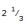Courses

# Races and Games

## 12 Questions MCQ Test General Knowledge | Races and Games

Description
This mock test of Races and Games for Current Affairs helps you for every Current Affairs entrance exam. This contains 12 Multiple Choice Questions for Current Affairs Races and Games (mcq) to study with solutions a complete question bank. The solved questions answers in this Races and Games quiz give you a good mix of easy questions and tough questions. Current Affairs students definitely take this Races and Games exercise for a better result in the exam. You can find other Races and Games extra questions, long questions & short questions for Current Affairs on EduRev as well by searching above.
QUESTION: 1

Solution:
QUESTION: 2

Solution:
QUESTION: 3

### In a 300 m race A beats B by 22.5 m or 6 seconds. B's time over the course is:

Solution:
QUESTION: 4
A and B take part in 100 m race. A runs at 5 kmph. A gives B a start of 8 m and still beats him by 8 seconds. The speed of B is:
Solution:
QUESTION: 5
A can run 224 metre in 28 seconds and B in 32 seconds. By what distance A beat B?
Solution:
QUESTION: 6

A istimes as fast as B. If A gives B a start of 80m,how long should the race course be so that both of them reach at the same time?

Solution:
QUESTION: 7
In a 500 m race, the ratio of the speeds of two contestants A and B is 3 : 4. A has a start of 140 m. Then, A wins by: B.
Solution:
QUESTION: 8
10. A and B take part in 100 m race. A runs at 5 kmph. A gives B a start of 8 m and still beats him by 8 seconds. The speed of B is:
Solution:
QUESTION: 9
In a 100 m race, A can give B 10 m and C 28 m. In the same race B can give C :
Solution:
QUESTION: 10

At a game of billiards, A can give B 15 points in 60 and A can give C to 20 points in 60. How many points can B give C in a game of 90?

Solution:
QUESTION: 11
In a race of 200 m, A can beat B by 31 m and C by 18 m. In a race of 350 m, C will beat B by:
Solution:
QUESTION: 12
In a 100 m race, A can beat B by 25 m and B can beat C by 4 m. In the same race, A can beat C by:
Solution:

• GAMES